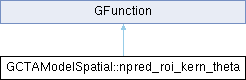GammaLib  1.7.0.dev
GCTAModelSpatial::npred_roi_kern_theta Class Reference

#include <GCTAModelSpatial.hpp>

Inheritance diagram for GCTAModelSpatial::npred_roi_kern_theta:## Public Member Functions

npred_roi_kern_theta (const GCTAModelSpatial *spatial, const GEnergy &energy, const GTime &time, const GCTAInstDir &roi_centre, const int &min_iter, const int &max_iter)

double eval (const double &theta)
Kernel for offset angle integration of spatial component. More...Public Member Functions inherited from GFunction
GFunction (void)
Void constructor. More...

GFunction (const GFunction &function)
Copy constructor. More...

virtual ~GFunction (void)
Destructor. More...

GFunctionoperator= (const GFunction &function)
Assignment operator. More...

## Protected Attributes

const GCTAModelSpatialm_spatial
Pointer to spatial component. More...

GEnergy m_energy
Energy. More...

GTime m_time
Time. More...

GCTAInstDir m_roi_centre
RoI centre. More...

int m_min_iter
Minimum number of Romberg iterations. More...

int m_max_iter
Maximum number of Romberg iterations. More...Protected Member Functions inherited from GFunction
void init_members (void)
Initialise class members. More...

void copy_members (const GFunction &function)
Copy class members. More...

void free_members (void)
Delete class members. More...

## Detailed Description

Definition at line 101 of file GCTAModelSpatial.hpp.

## Constructor & Destructor Documentation

 GCTAModelSpatial::npred_roi_kern_theta::npred_roi_kern_theta ( const GCTAModelSpatial * spatial, const GEnergy & energy, const GTime & time, const GCTAInstDir & roi_centre, const int & min_iter, const int & max_iter )
inline

Definition at line 103 of file GCTAModelSpatial.hpp.

## Member Function Documentation

 double GCTAModelSpatial::npred_roi_kern_theta::eval ( const double & theta )
virtual

Kernel for offset angle integration of spatial component.

Parameters
 [in] theta Offset angle from RoI centre (radians).

Computes

$K(\rho | E, t) = \sin \theta \times \int_{0}^{2\pi} B(\theta,\phi | E, t) d\phi$

where $$B(\theta,\phi | E, t)$$ is the spatial component of the background model for a specific observed energy $$E$$ and time $$t$$.

Implements GFunction.

Definition at line 499 of file GCTAModelSpatial.cpp.

## Member Data Documentation

 GEnergy GCTAModelSpatial::npred_roi_kern_theta::m_energy
protected

Energy.

Definition at line 118 of file GCTAModelSpatial.hpp.

Referenced by eval().

 int GCTAModelSpatial::npred_roi_kern_theta::m_max_iter
protected

Maximum number of Romberg iterations.

Definition at line 122 of file GCTAModelSpatial.hpp.

Referenced by eval().

 int GCTAModelSpatial::npred_roi_kern_theta::m_min_iter
protected

Minimum number of Romberg iterations.

Definition at line 121 of file GCTAModelSpatial.hpp.

Referenced by eval().

 GCTAInstDir GCTAModelSpatial::npred_roi_kern_theta::m_roi_centre
protected

RoI centre.

Definition at line 120 of file GCTAModelSpatial.hpp.

Referenced by eval().

 const GCTAModelSpatial* GCTAModelSpatial::npred_roi_kern_theta::m_spatial
protected

Pointer to spatial component.

Definition at line 117 of file GCTAModelSpatial.hpp.

Referenced by eval().

 GTime GCTAModelSpatial::npred_roi_kern_theta::m_time
protected

Time.

Definition at line 119 of file GCTAModelSpatial.hpp.

Referenced by eval().

The documentation for this class was generated from the following files: